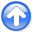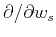First-break traveltime tomography with the double-square-root eikonal equationNext: Appendix C: Adjoint-state tomography Up: Li et al.: DSR Previous: Appendix A: Causal discretization

# Appendix B: Frechét derivative of DSR tomography

To derive the Frechét derivative, we start from equations 13 and 14. Applyingto both sides of equation 13 results in(35)

Analogously(36)

Inserting equations B-1 and B-2 into 14 and regrouping the terms, we prove equation 15(37)

where(38)

and(39)(40)

At the singularity of DSR eikonal equation, the operators,andtake simpler forms and can be derived directly from equation 3.First-break traveltime tomography with the double-square-root eikonal equationNext: Appendix C: Adjoint-state tomography Up: Li et al.: DSR Previous: Appendix A: Causal discretization

2013-10-16